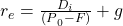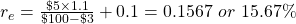Cost of Capital | IFT World
IFT Notes for Level I CFA® Program
IFT Notes for Level I CFA® Program

# Part 4

## 5. Flotation Costs

Floatation costs are the fees charged by investment bankers when a company raises external capital. There are two approaches to deal with floatation costs:

Approach 1: Incorporate flotation costs into the cost of capital. This will increase the cost of capital.For example, consider a company that has a current dividend of $5 per share, a current price of$100 per share and an expected growth rate of 10%. The cost of equity without considering floatation costs would be:If the floatation costs are 3% of the issuance, the cost of equity considering the floatation costs would be:However, the problem with this approach is that floatation costs are not an ongoing expense, they are a cost that the firm incurs at the start of the project. Hence, we should not be discounting all future cash flows at a higher cost of capital. The correct way to treat floatation costs is to use approach 2.

Approach 2: We adjust the initial cash flow by the amount of floatation costs. We do not adjust the discount rate.

Let’s say in the above example, the company raised $100,000 for a project by issuing new shares. The floatation costs would be 3% of$100,000 i.e. $3,000. In this approach we increase the initial cash outlay of the project to$103,000. The cost of equity, however, remains unchanged at 15.5%.

## 6. Methods in Use

In this reading, we saw several methods to estimate the cost of capital for a company or project. A survey of a large number of company CFOs to understand the methods they use to estimate the cost of capital revealed the following:

• The capital asset pricing model is the commonly used model. The single-factor capital asset pricing model is the most popular one.
• Few companies use the dividend cash flow model.
• Publicly traded companies were more likely to use the capital asset pricing model than private companies.
• Most companies used a single cost of capital across projects, while some used risk adjustments for individual projects.